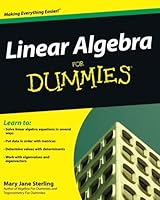# Linear Algebra For Dummies50 Reviews
2009-07-07
384 pages

## Book Description

Learn to:

• Solve equations in several ways
• Put data in order with matrices
• Determine with determinants
• Work with eigenvalues and eigenvectors

Your hands-on guide to real-world applications of linear

Does linear algebra leave you feeling lost? No worries —this easy-to-follow guide explains the how and the why of solving linear algebra problems in plain English. From matrices to vector spaces to linear transformations, you'll understand the key concepts and see how they relate to everything from genetics to nutrition to spotted owl extinction.

• Line up the basics — discover several different approaches to organizing numbers and equations, and solve of equations algebraically or with matrices
• Relate vectors and linear transformations — link vectors and matrices with linear combinations and seek solutions of homogeneous systems
• Evaluate determinants — see how to perform the determinant function on different sizes of matrices and take advantage of Cramer's rule
• Hone your skills with vector spaces — determine the properties of vector spaces and their subspaces and see linear transformation in action
• Tackle eigenvalues and eigenvectors — define and solve for eigenvalues and eigenvectors and understand how they interact with specific matrices

Open the book and find:

• Theoretical and practical ways of solving linear algebra problems
• Definitions of terms throughout and in the glossary
• New ways of looking at operations
• How linear algebra ties together vectors, matrices, determinants, and linear transformations
• Ten common representations of Greek letters
• Real-world applications of matrices and determinants

### Table of Contents

Part I: Lining Up the Basics of Linear Algebra
Chapter 1: Putting a Name to Linear Algebra
Chapter 2: The Value of Involving Vectors
Chapter 3: Mastering Matrices and Algebra
Chapter 4: Getting Systematic with Systems of Equations

Part II: Relating Vectors and Linear Transformations
Chapter 5: Lining Up Linear Combinations
Chapter 6: Investigating the Matrix Equation AX=b
Chapter 7: Homing In on Homogeneous Systems and Linear Independence
Chapter 8: Making Changes with Linear Transformations

Part III: Evaluating Determinants
Chapter 9: Keeping Things in Order with Permutations
Chapter 10: Determining Values of Determinants
Chapter 11: Personalizing the Properties of Determinants
Chapter 12: Taking Advantage of Cramer’s Rule

Part IV: Involving Vector Spaces
Chapter 13: Promoting the Properties of Vector Spaces
Chapter 14: Seeking Out Subspaces of a Vector Space
Chapter 15: Scoring Big with Vector Space Bases
Chapter 16: Eyeing Eigenvalues and Eigenvectors

Part V: The Part of Tens
Chapter 17: Ten Real-World Applications Using Matrices
Chapter 18: Ten (Or So) Linear Algebra Processes You Can Do on Your Calculator
Chapter 19: Ten Mathematical Meanings of Greek Letters

## Book Details

• Title: Linear Algebra For Dummies
• Author:
• Length: 384 pages
• Edition: 1
• Language: English
• Publisher:
• Publication Date: 2009-07-07
• ISBN-10: 0470430907
• ISBN-13: 9780470430903

## Book DownloadTry Audible and Get 2 Free Audiobooks »

Download LinkFormatSize (MB)Upload Date
Direct download (Recommended!)True PDF11.205/02/2019
Download from UsersCloudTrue PDF11.208/31/2018
How to Download? Report Dead Links & Get a Copy